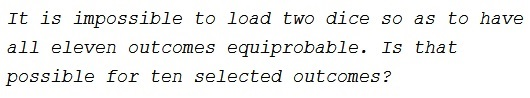### Problem### Solution

Let $\displaystyle P(x)=\sum_{k=1}^6p_ix^{i}$ be a probability generating function for the first die, $\displaystyle Q(x)=\sum_{k=1}^6q_ix^{i}$ that for the second. The polynomial coefficients are the "weights" for each of the indices. Let's set $p_1=0,$ meaning that the first die never come up with $1$ on top. Factor out $x^2$ from $P$ and ${x}$ from $Q:$

$P(x)=x^2P_0(x)=x^2(p_2+p_3x+p_4x^2+p_5x^3+p_6x^4),\\ Q(x)=xQ_0(x)=x(q_1+q_2x+q_3x^2+q_4x^3+q_5x^4+q_6x^5).$

Assuming that the outcomes $3,4,\ldots,12$ are equiprobable

\displaystyle\begin{align} P_0(x)Q_0(x)&=\frac{1}{10}(1+x+x^2+\ldots+x^9)\\ &=\frac{1}{10}\cdot\frac{x^{10}-1}{x-1}=\frac{1}{10}\frac{(x^5-1)(x^5+1)}{x-1}\\ &=\frac{1}{10}\cdot\frac{1+x+x^2+x^3+x^4}{5}\cdot\frac{1+x^5}{2}. \end{align}

Which means that there is a choice

$\displaystyle p_1=0,\,p_2,\,p_3,\,p_4,\,p_5,\,p_6=\frac{1}{5}\\ \displaystyle q_1=q_6=\frac{1}{2},\,q_2=q_3=q_4=q_5=0.$

The second die is easily created by taking a regular cube and placing numbers $1$ and $6$ on three faces each. Both of them can be simulated by a pair of regular icosahedra (each with 20 faces). One with ten faces bearing $1$ and ten faces bearing $6.$ The other will have numbers (or number dots) $2,$ $3,$ $4,$ $5,$ $6$ on four faces each.

### Acknowledgment

This is a continuation of an earlier problem and appeared in the Chapter Mathematical Creativity in Problem Solving (by Morray S. Klamkin) in In Eves' Circles (J. M. Anthony, editor, MAA 1994)